# 3: Ready for Prime Time

by Kirby Urner
First posted: Feb 16, 2000

In this section, we reintroduce odd versus even, and prime versus composite numbers -- already familiar ground to most students. The well-worn nature of these topics gives your studnets more of an opportunity to focus on the novel aspects of your presentation, including any historical background you may care to provide, or suggest that students research.

One of our goals is to continue building on concepts and skills already introduced in the previous two sections. Number series, sphere packing and Pascal's Triangle, as well as our highly synergetic combination of software tools -- the "dynamic duo" of Python + Povray -- will remain central to this Oregon Curriculum Network approach.

In the interactive session below, the iseven and isodd tests (defined at right) are being mapped to rows 5 and 6 of Pascal's Triangle, as returned by the prow function. 1 means true, 0 means false.

```>>> import series
>>> import primes
>>> series.prow(5)
[1L, 5L, 10L, 10L, 5L, 1L]
>>> map(primes.iseven,series.prow(5))
[0, 0, 1, 1, 0, 0]
>>> series.prow(6)
[1L, 6L, 15L, 20L, 15L, 6L, 1L]
>>> map(primes.iseven,series.prow(6))
[0, 1, 0, 1, 0, 1, 0]
```
 ```defiseven(n): """Return true if n is even.""" return n%2==0 def isodd(n): return not iseven(n) ```

Note that iseven uses the built-in Python operator: %. This is a binary operator, meaning it expects two arguments, arg1 and arg2, in this case using the syntax arg1 % arg2. This operator's job is to return the remainder after dividing arg1 by arg2. If dividing arg1 by 2 yields a remainder of 0, then arg1 must be even, odd otherwise.

 The code excerpt below (from packing.py) assigns a sphere color based on whether some test1 returns true or false. Then the sphere is written to a file. ``` if test1(i,j): myfile.sphcolor = "Orange" else: myfile.sphcolor = "Black" myfile.point(v2) ``` The test in question simply asks "is this entry in Pascal's Triangle an even number?" ```def test1(i,j): entry = series.pascal(i-1,j) return primes.iseven(entry) ```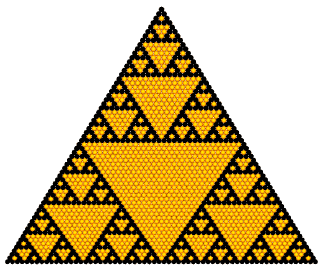Pascal's Triangle: Odd=Black, Even=Orange This fractal pattern, corresponding to even and odd entries in Pascal's Triangle, is also known as Sierpinski's Triangle, after Waclaw Sierpinski (1882-1969). Notice the camera is here looking straight into the (x,y)-plane -- a different viewing angle than in the previous section. Exactly where and how such alterations get made will depend on the task at hand, and student preference. Those students most comfortable with altering and reloading modules may find it convenient to alter the header method in Python, especially in cases where lots of pending graphical output is to be viewed from the same angle. On the other hand, in some situations, tweeking the Povray file on-the-fly, and re-rendering it, may be the most satisfying approach. Counting numbers are either even or odd. They are also either prime or composite, with the exception of 1 (some authors consider 1 prime). Primes cannot be divided by any number (except 1) without leaving a remainder. The composites are all products of primes. Test functions isprime and iscomposite will return 1 or 0 depending on whether a number is prime or not.
 ```>>> import primes >>> import series >>> primes.isprime(40058951) 1 >>> primes.iscomposite(20489) 1 >>> fibs = map(series.fibo,range(10)) >>> map(int,fibs) [0, 1, 1, 2, 3, 5, 8, 13, 21, 34] >>> map(primes.isprime,fibs) [0, 0, 0, 1, 1, 1, 0, 1, 0, 0] >>> fibs = map(series.fibo,range(41,44)) >>> fibs [165580141L, 267914296L, 433494437L] >>> map(primes.isprime,fibs) [0, 0, 1] >>> map(primes.isprime,series.prow(5)) [0, 1, 0, 0, 1, 0] ```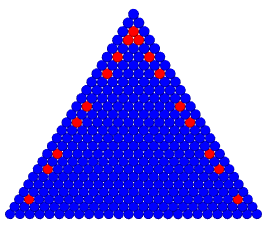Pascal's Triangle: Prime=Red, Not-Prime=Blue
 isprime has the job of adding primes to our list of consecutive primes. It only adds enough primes to determine whether the passed argument n is divisible. isprime depends on a simple "trial by division" algorithm. In trial by division, we divide a candidate prime by all primes up to some cut-off value. If we always get a remainder (i.e. no smaller prime divides it), the candidate gets added to the list, and so on. The candidate's 2nd root provides the cut-off, as any higher primes would necessarily pair with lower primes under this value. Trial by division is a "brute force" approach in that it fails to take advantage of many tests for primality which number theorists have devised over the centuries. This keeps us in the realm of smaller primes. As students become more aware of the limitations of such "brute force" strategies, their appreciation for more thoughtful and more powerful approaches may grow -- a lesson with applications in many walks of life besides mathematics. Two of these more "thoughtful tests", fermat and euler, have been included in the primes.py module. Encourage your students to play with these functions, to appreciate their applicability and limitations. The goal, for the purposes of this essay, is to anchor a sense of exactly what these tests do, leaving proofs or explanations for later. Just making the propositions of number theory comprehensible (e.g. "the Carmichael numbers are pseudoprimes which pass the fermat test for any base, and may be generated ad infinitum") is a worthy goal at this level, and should be a prerequisite for proving anything about their internal self-consistency. Students need answers to "what?" before "why?". The divtrial function returns true if a candidate proves to be prime: ```def divtrial(n): # trial by division to check whether a number is prime verdict = 1 # default is "yes, add to list" for i in primes: if (n%i == 0): # no remainder verdict = 0 # so we _don't_ want to add break if i*long(i) > n: # stop trying to divide by break # lower primes when p**2 > n return verdict ``` The erastosthenes function, based on the famous Sieve of Erastosthenes (276 BC-194 BC), starts with a list of 1s corresponding to 2 (the only even prime) and all odd integers through some maximum number N (the candidate primes), and with 0s in all even positions. Some programmers leave out the even positions entirely, to save on memory. Here's a schematic view: ``` Sieve: [ 0 0 1 1 0 1 0 1 0 1 0 1 0 1 0 1 ...] Position/Integer: [ 0 1 2 3 4 5 6 7 8 9 10 11 12 13 14 15 ...] ``` Find the first 1 in the sieve beyond position 2 (i.e. 3 -- marked with an asterisk), and, starting from the 2nd power of the corresponding integer (= 9), step through the sieve by twice its value (= 6), changing any 1s to 0s (see tic marks below). After this first iteration, your sieve will look like this: ``` * Sieve: [ 0 0 1 1 0 1 0 1 0 0 0 1 0 1 0 0 ...] Position/Integer: [ 0 1 2 3 4 5 6 7 8 9 10 11 12 13 14 15 ...] ^ ^ ``` Move to the next odd integer still with a corresponding 1 (i.e. 5), and repeat the operation, starting with 25 and stepping through by 10, again changing 1s to 0s. Keep looping through these steps. The algorithm is complete when the next odd integer to be considered exceeds the 2nd root of N. At this point, integers with corresponding 1s comprise the complete set of primes from 2 through N. Only the list of 1s and 0s, N, and the stepping number itself need be in memory until the sieving is finished, at which point the corresponding primes may be listed. Note: if you want to run any of this code, you should grab it from primes.py or download python101.zip (contains all the modules) as cutting and pasting from these web pages will pick up a lot of colorizing font tags which you would then have to remove. ```deferastosthenes(n): """ with suggestions from Ka-Ping Yee, John Posner and Tim Peters """ sieve = [0, 0, 1] + [1, 0] * (n/2) # [0 0 1 1 0 1 0...] prime = 3 # initial odd prime while prime**2 <= n: for i in range(prime**2, n+1, prime*2): sieve[i] = 0 # step through sieve by prime*2 prime += 1 + sieve[prime+1:].index(1) # next prime (2.0+ syntax) # filter includes corresponding integers where sieve = 1 return filter(lambda i, sieve=sieve: sieve[i], range(n+1)) ``` In a variant of the above, instead of starting with an array of 1s and 0s, we might use more memory and initialize with odd numbers from 3 to n, and then change them to 0 by successive sieving. Here is such an algorithm: ```defsieve(n): """ In-place sieving of odd numbers, adapted from code by Mike Fletcher """ candidates = range(3, n+1, 2) # start with odds for p in candidates: if p: # skip zeros if p*p>n: break # done for q in xrange(p*p, n+1, 2*p): # sieving candidates[(q-3)/2] = 0 return  + filter(None, candidates) #  + remaining nonzeros ``` The get2max function, used below, returns all primes up to and including the passed argument. Another function, get2nb, returns a list with a fixed number of consecutive primes (starting with 2). Both of these methods depend on the divtrial method, i.e. use trial by division. ```>>> import primes >>> primes.primes # primes initializes to   >>> primes.get2max(34) [2, 3, 5, 7, 11, 13, 17, 19, 23, 29, 31] >>> primes.get2max(45) # extending to higher primes [2, 3, 5, 7, 11, 13, 17, 19, 23, 29, 31, 37, 41, 43] >>> primes.get2max(10) # no new primes needed to answer [2, 3, 5, 7] >>> primes.primes # all primes computed so far are still on file [2, 3, 5, 7, 11, 13, 17, 19, 23, 29, 31, 37, 41, 43] >>> primes.erastosthenes(20)[2, 3, 5, 7, 11, 13, 17, 19] ``` The primes module contains a variable called primes outside of all function definitions, meaning it is scoped to the module itself, and persists as long as the module is in memory. As the user asks for higher and higher primes, all those found so far remain in primes, saving computation time. This technique saves time, by not throwing away already-computed primes, but means methods like get2max and get2nb have to pay attention to what primes are already out there, in order to decide how many, if any, to add. Here's the code for isprime: ```def isprime(n): # trial by division: divide n by primes up to maxnb = root(n) # return 0 if n is divisible # return 1 if n is prime nbprimes = 0 rtnval = 1 if n == 2: return 1 if n < 2 or iseven(n): return 0 maxnb = n ** 0.5 # 2nd root of n # if n < largest prime on file, check for n in list if n <= primes[-1]: rtnval = (n in primes) # if primes up to root(n) on file, run sieve (a prime test) elif maxnb <= primes[-1]: rtnval = sieve(n) else: rtnval = divtrial(i) # check divisibility by primes so far if rtnval==1: # now, if still tentatively prime... # start with highest prime so far i = primes[-1] # and add... i = i + 1 + isodd(i) # next odd number while i <= maxnb: if divtrial(i): # test of primehood primes.append(i) # append to list if prime if n%i==0: # if n divisible by latest prime rtnval = 0 # then we're done break i=i+2 # next odd number return rtnval ```
 Now that we have a function to generate primes in inventory, we can do other useful operations. For example we may list all prime factors of a composite number. My definition of getfactors function is recursive, and depends on having handy all primes up to the 2nd root of n. isprime, invoked at the outset, will ensure this list is complete. Again, given the "brute force" methods involved, this approach is not very practical for cracking big number with large prime factors.```defgetfactors(n): """Return list containing prime factors of a number.""" if isprime(n) or n==1: return [n] else: for i in primes: if not n%i: # if goes evenly n = n/i return [i] + getfactors(n) ```
 The getfactors method provides a basis for obtaining greatest common divisor (GCD) of two numbers. We might compare the prime factors of two arguments and return the product of all those common to both (if any). This would be the intersection of the two sets of primes. However, we really do not need complete prime factorizations of two numbers in order to determine their GCD, which is fortunate, as finding all the prime factors of a number is not necessarily an easy job -- is next to impossible for very large numbers, even using fast computers. In fact, it's the "uncrackability" of very large numbers (hundreds of digits) which serves as a basis for various encryption algorithms. The RSA algorithm, for example, starts by randomly generating a pair of hundred-digit primes and multiplying them together. If this operation were easily reversible, such that we could get the original pair of prime factors when given their product, then RSA encryption would not be secure. But to date we know of no practical way to factor such huge products (plus RSA may be crackable by other means, as yet undiscovered or unpublished). A far more efficient way of find the GCD traces back to Euclid (325 BC - 265 BC) and possibly before, and is called Euclid's Algorithm in many texts. This is one of the oldest algorithms on record. The Python version is quite compact (was this originally from Guido?):
 ```defgcd(a,b): # return greatest common divisor # using Euclid's Algorithm while b: a, b = b, a % b return a ``` The idea here is that if neither a nor b is itself the gcd, then the next step is to find a number which divides evenly into both b and the remainder (a % b) -- and that means we're looking for a gcd again. The remainder gets smaller, and ultimately reaches 0 (perhaps from dividing by 1), at which point we have our answer. For example, in looking for gcd(30,18), we find a remainder of 12. So the gcd must divide 18 and 12 meaning we're looking for gcd(18,12). The next remainder is 6, so now we want the gcd of 12 and 6. At this point, a remainder of 0 gets us out of the the loop, and 6 is the final answer. This function could also be written recursively: ```def gcd(a,b): if b==0: return a else: return gcd(b,a%b) ```
 The lcm method returns the lowest common multiple of two numbers using the following logic: lcm(a,b) = all factors of both, divided by factors common to both: gcd(a,b) = (a*b)/gcd(a,b). ``` deflcm(a,b): # union of all factors (a*b), # divided by gcd (factors in common) return (a*b)/gcd(a,b) >>> primes.getfactors(5985) # above formula shows factors intermultipied [3, 3, 5, 7, 19] >>> primes.getfactors(804) [2, 2, 3, 67] >>> primes.getfactors(1034) [2, 11, 47] >>> primes.gcd(1034,804) # gcd = product of primes in common 2 >>> primes.gcd(10,105) 5 >>> primes.gcd(48,60) 12 >>> primes.lcm(48,60) # lcm = product of factors in union of factor sets 240 >>> primes.lcm(1034,804) 415668 >>> series.fibo(63) # 63rd Fibonacci number 6557470319842L >>> primes.getfactors(series.fibo(63)) # prime factors of 63rd fib. no. [2, 13, 17, 421, 35239681L] >>> fibs = map(series.fibo,range(1,10)) # list of 9 fibonacci numbers >>> fibs [1L, 1L, 2L, 3L, 5L, 8L, 13L, 21L, 34L] >>> primes.LCM(fibs) # LCM (uppercase) accepts lists, as does GCD() 185640L >>> primes.getfactors(18446744073709551615L) # pretty fast [3, 5, 17, 257, 641, 65537, 6700417L] >>> primes.getfactors(18446744073709551597L) # very hard to factor [ eventually gives a memory error -- more than this program can handle ] ``` With getfactors in place, we may define a simple function telling us how many integers less than n are are relatively prime to n, meaning they have no factors in common. Two integers are relatively prime if they have no common divisor other than 1 (gcd=1). For example, 1,3,7 and 9 are relatively prime to 10, whereas 2, 4 and 5 are not. Leonhard Euler (1707-1783) proved the product n(1-1/F0)(1-1/F1) ... (1-1/Fn) gives the number of relative primes for n, where F0, F1... Fn are n's prime factors. The idea is to cancel every 2nd, 3rd , 5th, 7th number and so on, with (1-1/Fn) being the fraction of integers remaining per the nth cancelation. We only want to use each prime factor once in this product, no matter how many times it occurs in the prime factorization. Therefore the phi (or "totient") function keeps a list of factors already used, and skips any reoccurances. ```defphi(n): """ Return number of integers < n relatively prime to n.""" product = n used = [] for i in getfactors(n): if i not in used: # use only unique prime factors used.append(i) product = product * (1 - 1.0/i) return int(product) ``` You might want to take this opportunity to point out to students that this use of phi(n) -- for the number of prime residuals < n -- is by convention, yet is of course different from the golden mean or any function for computing this value. The Python concepts of namespaces is relevant in this connection: every module has its own dictionary of key terms, and prefixing the module name (as we have been doing), helps resolve any ambiguities that might arise from such multiple meanings. A namespace is a context. Notice that all integers less than a prime are relatively prime, i.e. phi(p), where p is prime, returns an answer of p-1. Euler's Theorem states that if and only if a and b are relatively prime (i.e. gcd(a,n) == 1), then pow(a,phi(n),n) == 1 i.e. pow(a,phi(n))/n has a remainder of 1. Note that whereas phi(n) always satisfies this condition, some smaller divisor of phi(n) may actually be the minimal exponent for which the remainder is unity. The thinking here is that integers relatively prime to n have relatively prime remainders (i.e. each relative prime mod n = some relative prime), and that any n-relative prime multiplied by all possible relative prime remainders will result in the same remainders all over again. So a * (all n-relative primes) mod n = (n-relative primes) mod n, and canceling identical terms on both sides leaves a^phi(n) = 1 mod n. You might want to explore this assertion that "any n-relative prime multiplied by all possible relative prime remainders will result in the same remainders all over again" by actually testing it for special case b and n, where gcd(b,n)=1. Collect all integers nrp < n which are n-relative primes, and compute (b * nrp) mod n for all of them. You should get back the same list of nrps (n-relative primes), perhaps in a different order. Here's some code: ```defrelprimes(n,b=1): """ List n-relative primes, then... List the remainders after dividing n by the product of each n-relative prime and some relative prime b """ relprimes = [] for i in range(1,n):ee if gcd(i,n)==1: relprimes.append(i) print " n-rp's: %s" % (relprimes) # multiply all relative primes < n by b (also an n-rp) relprimes = map(operator.mul,[b]*len(relprimes),relprimes) # list all the above products mod n newremainders = map(operator.mod,relprimes,[n]*len(relprimes)) print "b * n-rp's mod n: %s" % newremainders ``` Usage: ```>>> import primes >>> primes.relprimes(12,5) n-rp's: [1, 5, 7, 11] b * n-rp's mod n: [5, 1, 11, 7] >>> primes.relprimes(30,7) n-rp's: [1, 7, 11, 13, 17, 19, 23, 29] b * n-rp's mod n: [7, 19, 17, 1, 29, 13, 11, 23] ``` And here's some code for testing Euler's theorem. If (a,n) have a gcd of 1, i.e. are relative primes, then this function should always return 1. ```deftesteuler(a,n): """Test Euler's Theorem""" a = long(a) if gcd(a,n)<>1: print "(a,n) not relative primes" else: print "Result: %s" % pow(a,phi(n),n) ``` Note this use of Python's built-in pow() function, which optionally takes a 3rd argument. pow(a,b) is equivalent to a**b whereas pow(a,b,n) is symbolically equivalent to (a**b)%n -- but is operationally much faster, owing to internally-implemented shortcuts (so use it instead). Pierre de Fermat (1601-1655) proved that any prime must satisfy the condition that for any integer a not a multiple of p, a to the power of p-1, divided by p, has a remainder of 1. In Python notation, we might write: pow(b,p-1,p) == 1, provided of course that gcd(b,p)=1. We call this Fermat's Little Theorem" (the "big one" being the more famous "last" one, and proved by Andrew Wiles in 1995). This theorem actually follows from Euler's (above), as phi(p) = p-1, i.e. all numbers less than a prime are relatively prime, by definition. Setting base b=2 creates a useful test for composite numbers. If a number fails this test, it's definitely not a prime. If it passes, it may be prime. Candidate primes may be further tested with higher bases, as a composite which passes fermat for base 2 may fail for some other base. For example, 341 passes when b=2, but fails when b=3: ```>>> primes.fermat(341,2) 1 >>> primes.fermat(341,3) 0 ``` However a few composites pass the fermat test regardless of our selection for b -- these are the Carmichael numbers, named for Robert Daniel Carmichael (1879-?), of which 561 is the first. And there's no theoretical upper limit on how many we might generate. Euler was also able to prove a stronger version of the Fermat's Little Theorem, which sieves out even more composites than Fermat's test: if p is prime, then pow(b,(p-1)/2,p) = jacobi(b,p). The jacobi function, named for Carl Gustav Jacob Jacobi (1804 - 1851), returns either 1,0 or -1. We say a mod n = -1 if the remainder of a/n is n-1 -- something to test for in the euler function. Again, base b is a variable. ```>>> plist = primes.sieve(60)[1:] # get primes below 60, slicing off 2 >>> plist[3, 5, 7, 11, 13, 17, 19, 23, 29, 31, 37, 41, 43, 47, 53, 59] >>> map(primes.fermat,plist) # fermat test true for all primes [1, 1, 1, 1, 1, 1, 1, 1, 1, 1, 1, 1, 1, 1, 1, 1] >>> map(primes.euler,plist) # euler test true for all primes [1, 1, 1, 1, 1, 1, 1, 1, 1, 1, 1, 1, 1, 1, 1, 1] >>> map(primes.phi,plist) # phi(p)=p-1 relative primes < n [2, 4, 6, 10, 12, 16, 18, 22, 28, 30, 36, 40, 42, 46, 52, 58] >>> primes.fermat(561,2) # 561 passes fermat test for base 2... 1 >>> primes.fermat(561,3) # passes for base 3 too, all bases in fact 1 >>> primes.euler(561,2) # 561 passes the Euler test, base 2 1 >>> primes.euler(561,3) # but Euler test base 3 finally finds it out 0 >>> primes.getfactors(561) # 561 is composite, is the lowest Carmichael [3, 11, 17] >>> primes.divtrial(561) # ...as the divtrial function discovers 0 >>> primes.gcd(8,15) # 8 and 15 are relatively prime 1 >>> pow(15,primes.phi(8),8) # testing Euler's theorem 1 >>> pow(8,primes.phi(15),15) # ditto (reversing a and b) 1 >>> primes.gcd(10,25) # 10 and 25 are not relatively prime 5 >>> pow(10L,primes.phi(25),25) # as a consequence, the remainder is not 1 0L ```
 We may also revisit Goldbach's Conjecture, as yet unproved, taking advantage of the fact that it has been verified for all numbers within the computational range of student computers. Christian Goldbach (1690-1764) surmized that any even number could be written as the sum of two primes, and any odd number as the sum of three primes. The goldbach function returns such 2- and 3-term sums, turning odd number cases into even ones simply by taking 3 for one of the prime terms. The algorithm used in the included module is computationally expensive in that it invokes get2max, which finds all primes up to and including the passed argument -- then discovers a sum working down the list from the primes closest to your input. ```>>> import primes >>> primes.goldbach(46798) [29, 46769] >>> primes.goldbach(50901) [3, 5, 50893] >>> primes.goldbach(80017) [3, 17, 79997] >>> map(primes.goldbach,range(100,106)) [[3, 97], [3, 19, 79], [5, 97], [3, 3, 97], [3, 101], [3, 5, 97]] ```
 The version of Pascal's Triangle shown above, with primes highlighted in red, the rest in blue, is somewhat boring to look at. The primes all occur in the next-to-last and next-to-first columns. Thanks to Jim Nugent's essay on Pascal's Tetrahedron, I learned the "prime scan" test will yield a much more interesting pattern if we color code the spheres based on whether an entry is: prime one greater than a prime number one less than a prime number between two primes (both one greater and one less) none of the above Here's the function I used to test for the above conditions: ```def testpass3(i,j): entry = series.pascal(i,j) rtnval=0 if primes.isprime(entry): rtnval = 1 else: neg1 = primes.isprime(entry-1) pos1 = primes.isprime(entry+1) if neg1: rtnval = 2 if pos1: rtnval = 3 if neg1 and pos1: rtnval = 4 # overrides previous values return rtnval ```
 pascalpack contains the following code fragment, which runs the above testpass3 and color-codes accordingly: ``` for j in range(i): rtnval = testpass3(i-1,j) if rtnval == 1: myfile.sphcolor = "Orange" elif rtnval == 2: myfile.sphcolor = "Blue" elif rtnval == 3: myfile.sphcolor = "Green" elif rtnval == 4: myfile.sphcolor = "Red" else: myfile.sphcolor = "Black" myfile.point(v2) ```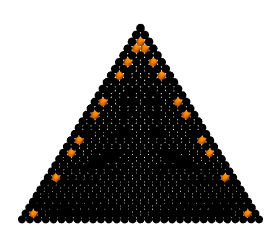Pascal's Triangle: Prime=Orange, Prime+1=Blue, Prime-1=Green, Between = Red, Rest = Black We can play the same game with Pascal's Tetrahedron. Entries climb to very high values rather quickly, and the algorithms provided in python101.zip bog down in trying to reach a verdict on the primehood of the giant numbers. Other algorithms might overcome these limitations. Using Python's anydbm module to save primes to the hard drive might take us beyond present horizons -- at a slow pace. Other short-cuts to determine primehood more quickly might also be incorporated -- a whole branch of mathematics and computer science has grown up around finding solutions to such problems.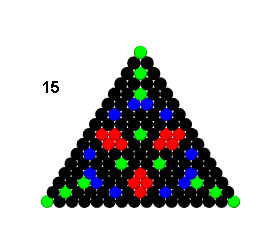Pascal's Tetrahedron: same coloring rules Note: level numbers start w/ apex as 0
 For further reading: Section 1: Getting Serious about Series Section 2: Getting Inventive with Vectors Section 4: Random Walks in the Matrix The Prime Pages Lesson Plan using Fermat's Test (note erratum, further explanations of erastosthenes code) More on gcd algorithm on edu-sig@python.org A fancier gcd algorithm (Python by Tim Peters) More on Euler's Phi function (math-teach elist) Number Theory links (including lots about primes) at Kevin Brown's MathPages Clubhouse Crypto (cryptography makes use of primes and number theory) python101.zip -- Python modules for this essay (right-click to download) Last Updated: Feb 5, 2001 A source for this section: Midhat Gazalé, Number from Ahmes to Cantor (Princeton University Press, 2000) -- see Amazon.com A heavier duty primes program for the Mac Background: On using big numbers to open new horizons in K-12 (Dec, 2000) Background: relevant postings to AMTE listserv (April, 2000) Background: Sieve of Erastosthenes thread on edu-sig@python.org Computer Language Resources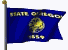Home Page